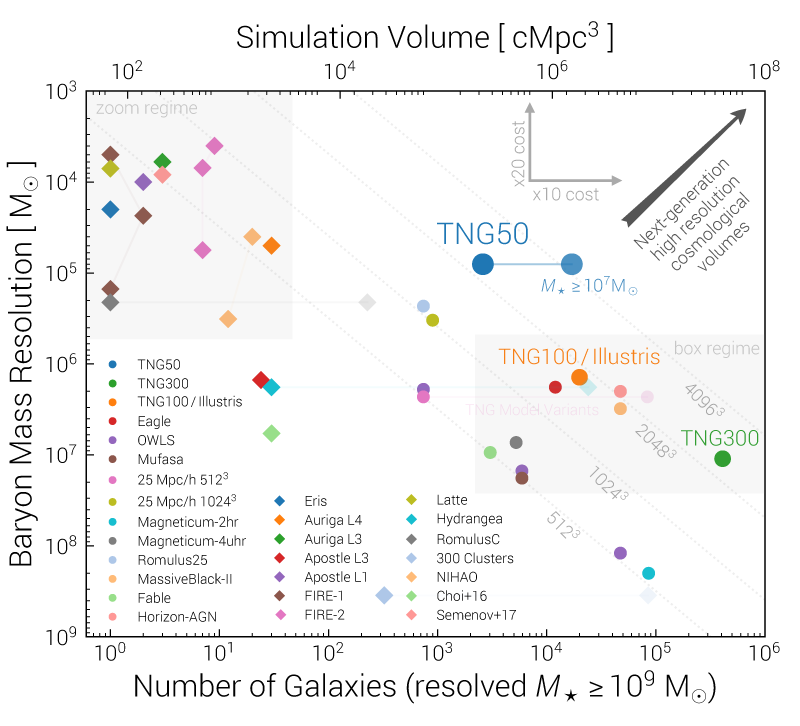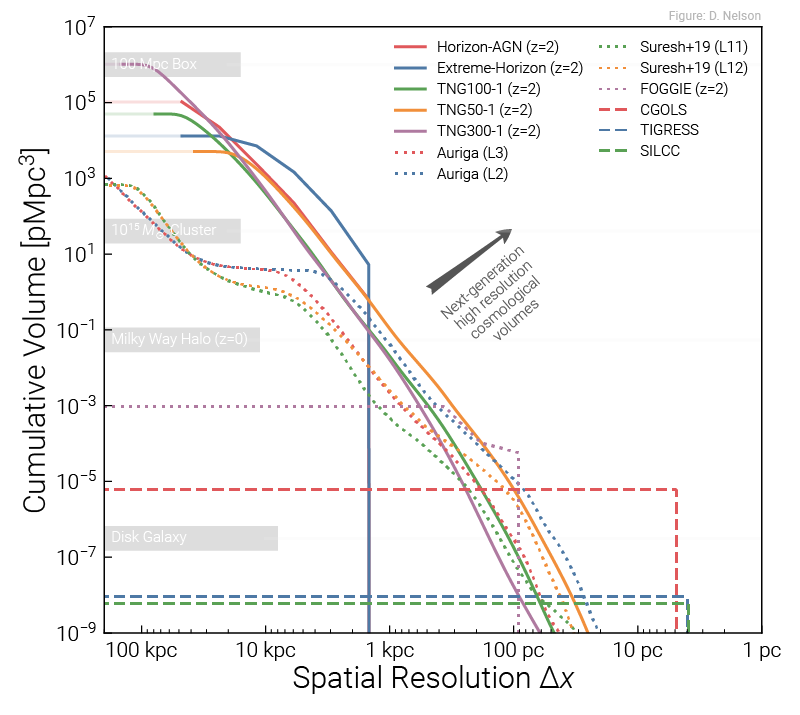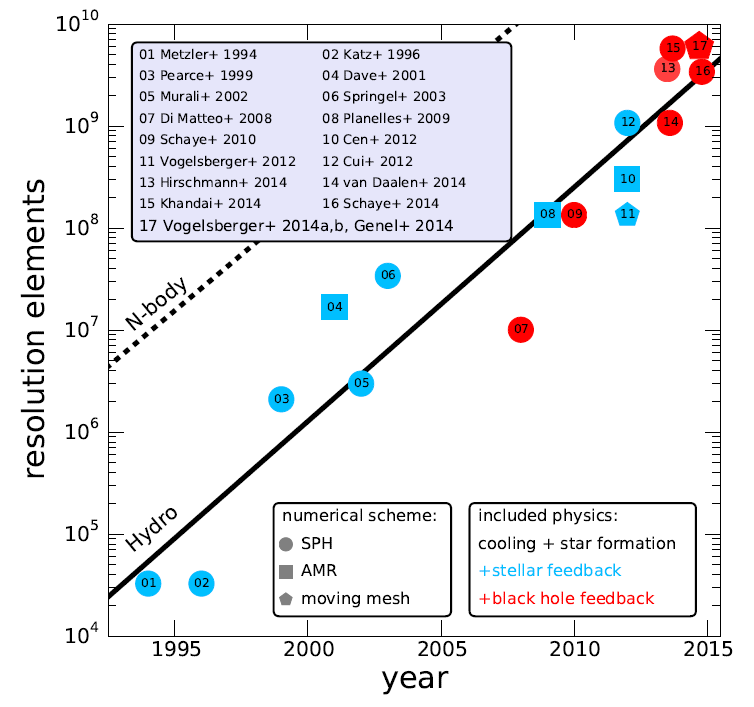There are many cosmological hydrodynamical simulations which are continually improving in terms of their volume, resolution, and physical modeling. Here we maintain a database of these numerical projects, along with their key characteristics for comparison. We also provide references and links to the figures and publications where a number of common galaxy population statistics and scaling relations have been presented. The availability of public data, or not, is noted.

We include simulations which have been evolved to $z \sim 0$, as opposed to those which stop substantially earlier, such as reionization projects. We also restrict this list to "full-box" periodic volumes, and not smaller zoom samples, as well as simulations with a reasonably high resolution such that galaxies can be studied ($M_{\rm DM} \lesssim 10^8 M_\odot$).

Since all simulations below broadly aim to reproduce the diversity of the galaxy population, they typically include models for star formation, stellar evolution and heavy element production, radiative (metal) cooling, stellar feedback, and supermassive black hole feedback. The details and sophistication of these models differ between simulations, as do their results and outcomes.

### Simulation List

Show secondary/small runs:
 Metadata Resolution References and Data Results SimulationName SimulationFamily Year Code Box Size $[\rm{cMpc}]$ $N_{\rm baryon}^\dagger$ $N_{\rm DM}$ $M_{\rm baryon}^\ddagger$$[\rm{M}_\odot] M_{\rm DM}$$[\rm{M}_\odot]$ $\epsilon_{\rm DM}$$[\rm{kpc}] \Delta x_{\rm gas,SF}$$[\rm{kpc}]$ $z_{\rm final}$ SimulationReference(s) MethodsReference(s) Website Public Data? Public Model? SMF $z=0$ SMF $z>0$ SMHM $z=0$ SMHM $z=1$ Cosmic SFRD Tully-Fisher Galaxy Colors Stellar Ages Stellar MZR Gas MZR SFMS Quenched Fraction Galaxy $f_{\rm H2}$ Halo $f_{\rm baryon,gas}$ Stellar Size $M_{\rm BH} - \sigma$ $M_{\rm BH} - M_{\star}$ $\Phi(L_{\rm AGN})$ Illustris Illustris 2014 AREPO $106.5$ $1820^3$ $1820^3$ $1.3 \times 10^6$ $6.3 \times 10^6$ $0.7$ $0.7$ 0 Vogelsberger+14 Genel+14 Sijacki+15 - - - - - Magneticum 2hr Magneticum 2014 GADGET-3 $500$ $1584^3$ $1584^3$ $2.0 \times 10^8$ $9.8 \times 10^8$ $5.3$ ? 0 Hirschmann+14 ? - - - - - - - - - - - - - - Magneticum 4uhr Magneticum 2014 GADGET-3 $68.2$ $576^3$ $576^3$ $1.4 \times 10^7$ $5.1 \times 10^7$ $2.0$ ? 0 Hirschmann+14 ? - - - - - - - - - - - Eagle Eagle 2015 GADGET-3 $100$ $1504^3$ $1504^3$ $1.8 \times 10^6$ $9.7 \times 10^6$ $0.7$ $1.1$ 0 Schaye+15 - - - - - Eagle25 Eagle 2015 GADGET-3 $25$ $752^3$ $752^3$ $2.3 \times 10^5$ $1.2 \times 10^6$ $0.35$ ? 0 Schaye+15 Crain+15 ? MassiveBlack-II MassiveBlack 2015 GADGET-3 $142.7$ $1792^3$ $1792^3$ $3.1 \times 10^6$ $1.6 \times 10^7$ $2.6$ ? 0 Khandai+15 ? - - - - - - - - - - - - - - - HorizonAGN HorizonAGN 2015 RAMSES $142$ ? $1024^3$ --- $8.0 \times 10^7$ ? $1.0$ 0 Dubois+14 ? - - - - - - - - - - - - Romulus25 Romulus 2016 ChaNGa $25$ $\sim 1100^3$ $\sim 1100^3$ $2.1 \times 10^5$ $3.4 \times 10^5$ $0.25$ ? 0 Tremmel+16 ? - - - - - - - - - - - - - MUFASA MUFASA 2016 GIZMO $73.5$ $512^3$ $512^3$ $1.8 \times 10^7$ $9.6 \times 10^7$ $0.74$ ? 0 Dave+16 ? - - - - - - - - - - TNG100 IllustrisTNG 2017 AREPO $110.7$ $1820^3$ $1820^3$ $1.4 \times 10^6$ $7.5 \times 10^6$ $0.74$ $0.7$ 0 Springel+18, Pillepich+18, Nelson+18, Marinacci+18, Naiman+18 , - - - TNG300 IllustrisTNG 2017 AREPO $302.6$ $2500^3$ $2500^3$ $1.1 \times 10^7$ $5.9 \times 10^7$ $1.5$ $1.4$ 0 Springel+18, Pillepich+18, Nelson+18, Marinacci+18, Naiman+18 , - - - - - - - - - FABLE FABLE 2018 AREPO $58.9$ $512^3$ $512^3$ $9.4 \times 10^6$ $5.0 \times 10^7$ $3.5$ ? 0 Henden+18 ? - - - - - - - - - - - - - Simba100 Simba 2019 GIZMO $147.1$ $1024^3$ $1024^3$ $1.8 \times 10^7$ $9.6 \times 10^7$ $0.74$ $0.53$ 0 Dave+19 ? - - - - - - Simba50 Simba 2019 GIZMO $73.5$ $1024^3$ $1024^3$ $2.3 \times 10^6$ $1.2 \times 10^7$ $0.37$ ? 1 Dave+19 ? TNG50 IllustrisTNG 2019 AREPO $51.7$ $2160^3$ $2160^3$ $8.5 \times 10^4$ $4.5 \times 10^5$ $0.29$ $0.28$ 0 Nelson+19, Pillepich+19 , - - - - - - - - - - - - - - NewHorizon NewHorizon 2020 RAMSES $16$ ? $\sim 470^3$ --- $1.2 \times 10^4$ ? $0.04$ 0 Dubois+21 ? - - - - - - FIREbox FIREbox 2022 GIZMO $22.1$ $1024^3$ $1024^3$ $6.3 \times 10^4$ $3.4 \times 10^5$ $0.08$ $0.01$ 0 Feldmann+22 - - - - - - - - MillenniumTNG MillenniumTNG 2022 AREPO $740$ $4320^3$ $4320^3$ $3.1 \times 10^7$ $1.7 \times 10^8$ $3.7$ $1.1$ 0 Hernandez-Aguayo+22, Pakmor+22 , - - - - - - - - - - -

### Simulation Code List

 Name Year Released Simulation(s) Run Hydro Method Gravity Method Reference(s) Public Code RAMSES 2002 HorizonAGN, NewHorizon Adaptive Mesh Refinement (AMR) Iterative Multigrid Teyssier+2002 GADGET-2/3 2005 EAGLE, MassiveBlack-II, Magneticum Smoothed Particle Hydrodynamics (SPH) TreePM Springel+2005 AREPO 2009 Illustris, IllustrisTNG, FABLE, MTNG Moving Voronoi Mesh (MM) TreePM Springel+2009 GIZMO 2014 Mufasa, Simba, FIREbox Meshless Finite Mass, Volume (MFM/MFV) TreePM Hopkins+2017 ChaNGa 2015 Romulus25 Smoothed Particle Hydrodynamics (SPH) Tree Menon+2015 SWIFT 2018 --- Smoothed Particle Hydrodynamics (SPH), MFM/MFV FMM-PM Schaller+2018 GADGET-4 2020 --- Smoothed Particle Hydrodynamics (SPH) FMM-PM, TreePM Springel+2020

### Notes

• $N_{\rm baryon}^\dagger$ - the initial number of gas particles/cells in Lagrangian type simulations, some fraction of which will be converted into stars at late times. For grid/Eulerian simulations, corresponds to the total number of leaf cells at $z=0$.
• $M_{\rm baryon}^\ddagger$ - if constant, the gas particle mass. If variable, the target/average gas cell mass for Lagrangian type simulations. For grid/Eulerian simulations, this value is not comparable and is omitted.

### Comparison Figures

Data like above has been combined to produce various comparison figures, and several are collected here.

(1) The Nelson et al. (2019) comparison of cosmological volumes and zooms, in terms of mass resolution and galaxy number statistics:(2) A comparison of spatial resolution, in terms of the total volume (y-axis) simulated at a given resolution $\Delta x$ or better (x-axis). Periodic boxes (solid) are compared against individual zooms and CGM-refined zooms (dotted) and idealized small-scale simulations (dashed):(3) The Genel et al. (2014) comparison of cosmological hydrodynamical simulations, in terms of the total number of resolution elements (particles), versus year: